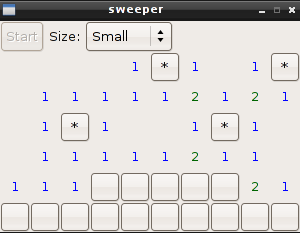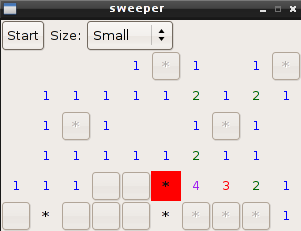2010-01-21
Sometimes, some things sort of get stuck in my head. The most recent concept to get stuck in my head was the number of elements in immediate proximity to a specific element in a grid. It started with two implementations of the Game of Life, one written in Vala using SDL and one written in Python using Clutter.

Do you know what else deals with the relationship of elements in a grid? MineSweeper!

MineSweeper happens to be one of my favorite computer games. At this point, it should be noted that there are few implementations of minesweeper for Linux. Of the two versions that I found, one requires Gnome and one Required KDE. Seriously? Why does a simple game need to require Gnome or KDE libs? Oh well, I guess I'll just have to write my own.

Enter the Vala:
```/*
compile with:
valac --pkg gtk+-2.0 main.vala -o sweeper
*/
using Gtk;
using Gdk;

public class Cell:EventBox
{
signal void flip_cell();
signal void flag_cell();
public int row;
public int col;
private Button button;
private Label label;
const string ONE= "blue";
const string TWO= "dark green";
const string THREE= "red";
const string FOUR= "purple";
const string FIVE= "orange";
const string SIX= "yellow";
private Color red;
construct
{
button = new Button();
//box = new Box();
}
public Cell(int r, int c)
{
Color.parse("red",out red);
row=r;
col=c;
//create the cell
button.set_relief(ReliefStyle.HALF);
button.event.connect( (obj,event)=> { parse_event(event); } );
//box.pack_start(button, true, true, 0);
label=new Label(null);
set_size_request(30,30);
}
public bool parse_event(Gdk.Event event)
{

if(event.button.type == Gdk.EventType.BUTTON_PRESS)
{
if (event.button.button==1) // && event.button.button<=3)
{
flip_cell();
}else if( event.button.button==3 )
{
flag_cell();
}
return true;
}else{
return false;
}
}
public void flag()
{
button.set_label("*");
}
public void end_flag()
{
if(button.get_label()!="*")
{
show_label("*");
}
}

public void show_label(string str)
{
string color;
switch(str)
{
case "1":
color = ONE;
break;
case "2":
color = TWO;
break;
case "3":
color = THREE;
break;
case "4":
color = FOUR;
break;
case "5":
color = FIVE;
break;
case "6":
color = SIX;
break;
/*case "7":
color = SEVEN;
break; */
default:
color = "black";
break;
}
label.set_markup("<span foreground=\"%s\">%s</span>".printf(color,str)      );
remove(button);
label.show();
}
{
modify_bg(StateType.NORMAL, red );
label.set_markup("<span background=\"red\" font_weight=\"heavy\">*</span>");
remove(button);
//box.pack_start(label,true,true,0);
label.show();
}

public void disable()
{
button.set_state(Gtk.StateType.INSENSITIVE);
}
}
public class Application:Gtk.Window
{
MainLoop loop;
bool[,] mineArray;
bool[,] flippedArray;
private Button startButton;
private ComboBox levelCombo;
private Table cellTable;
private int rows {get;set;}
private int cols {get;set;}
private int mines;
private Cell[,] cells;
private VBox vbox;
private bool game_playing;
private bool game_needs_new_table;
//define the small/medium/large values
const int small_rows=6;
const int small_cols=10;
const int small_mines=10;
const int medium_rows=10;
const int medium_cols=16;
const int medium_mines=26;
const int large_rows=12;
const int large_cols=20;
const int large_mines=40;
private int required_flips;
private int flip_count;
construct
{
type=Gtk.WindowType.TOPLEVEL;
set_resizable(false);
game_playing=false;
game_needs_new_table=false;
//we default to small
rows = small_rows;
cols = small_cols;
mines = small_mines;
}

public Application()
{
destroy.connect(quit);
vbox = new VBox(false,0);
HBox hbox = new HBox(false,0);
startButton = new Button.with_label("Start");
levelCombo = new ComboBox.text();
levelCombo.append_text("Small");
levelCombo.append_text("Medium");
levelCombo.append_text("Large");
//levelCombo.append_text("Custom");
levelCombo.set_active(0);
levelCombo.changed.connect( level_changed );

Label dif = new Label("Size:");
startButton.clicked.connect( start_game );
hbox.pack_start(startButton,false,false,0);
hbox.pack_start(dif,false,false,0);
hbox.pack_start(levelCombo,false,false,0);
hbox.set_spacing(5);
vbox.pack_start(hbox,false,false,0);
show_all();
//create the first table
new_table();
cellTable.set_sensitive(false);
}

private void level_changed()
{
string val = levelCombo.get_active_text();
switch(val)
{
case "Small":
rows = small_rows;
cols = small_cols;
mines = small_mines;
break;
case "Medium":
rows = medium_rows;
cols = medium_cols;
mines = medium_mines;
break;
case "Large":
rows = large_rows;
cols = large_cols;
mines = large_mines;
break;
}
game_needs_new_table=true;
start_game();
//we should probably resize the window
}

public void start_game()
{
if(game_needs_new_table)
{
new_table();
}
game_playing=true;
//disable the start button
startButton.set_sensitive(false);
cellTable.set_sensitive(true);
}
public void end_game()
{
//disable unflipped cells
for(int r=0; r<rows; r++)
{
for(int c=0; c<cols; c++)
{
if(!flippedArray[r,c])
{
//if this is a mine, mark it as such
if(mineArray[r,c])
{
cells[r,c].end_flag();
}
cells[r,c].disable();
}
}
}
game_needs_new_table=true;
//enable the start button
game_playing = false;
startButton.set_sensitive(true);
}

public void new_table()
{
vbox.remove(cellTable);
cellTable = make_table();
vbox.pack_start( cellTable,true,true,0 );
cellTable.show_all();
}

private Table make_table()
{
//reset the required flips
required_flips = rows*cols - mines;
//reset the flip_count
flip_count=0;
mineArray = new bool[rows,cols];
flippedArray = new bool[rows,cols];
cells = new Cell[rows,cols];
Table table = new Table(rows,cols,true);
for( int r=0;r<rows;r++ )
{
for ( int c=0; c<cols; c++ )
{
flippedArray[r,c]=false;
mineArray[r,c]=false;
cells[r,c] = new Cell(r,c);
cells[r,c].flip_cell.connect( (cell)=>{ flip_cell(cell); } );
cells[r,c].flag_cell.connect( (cell)=>{ flag_cell(cell); } );
//add the cell to the table lrtb
table.attach(cells[r,c],c,c+1,r,r+1,AttachOptions.FILL ,AttachOptions.FILL ,0,0);
}
}
//make the mines
int minecount=0;
int randomrow;
int randomcol;
Rand rand = new Rand();
while(minecount<mines)
{
//pick a random row
randomrow= rand.int_range(0,rows);
//pick a random col
randomcol = rand.int_range(0,cols);
if(!mineArray[randomrow,randomcol])
{
mineArray[randomrow,randomcol]=true;
minecount++;
}
}
return table;
}

public void flag_cell( Cell c )
{
c.flag();
}

private void flip_cell(Cell c)
{
//consider this flipped
flippedArray[c.row,c.col]=true;
if(mineArray[c.row,c.col])
{
end_game();
}else{
detect_neighboring_mines(c.row,c.col);
flip_count+=1;
if(flip_count==required_flips)
{
//we did it!
end_game();
}
}
}
private void detect_neighboring_mines(int r, int c)
{
string label="";
int mine_count = 0;
int r_min = r-1;
int r_max = r+1;
int c_min = c-1;
int c_max = c+1;
if(r==0)
{
r_min=r;
}
else if (r == rows-1)
{
r_max=r;
}
if ( c==0 )
{
c_min=c;
}
else if (c == cols-1)
{
c_max=c;
}
int tr = r_min;
int tc = c_min;
while(tr <= r_max)
{
tc = c_min;
while(tc <= c_max)
{
if ( tr!=r || tc!=c )
{
if ( mineArray[tr,tc])
{
mine_count+=1;
}
}
tc+=1;
}
tr+=1;
}
if(mine_count>0)
{
label = mine_count.to_string();
}else{
expand_from_empty_cell(r,c);
}
cells[r,c].show_label( label );
}

private void expand_from_empty_cell(int r, int c)
{
int r_min = r-1;
int r_max = r+1;
int c_min = c-1;
int c_max = c+1;
if(r==0)
{
r_min=r;
}
else if (r == rows-1)
{
r_max=r;
}
if ( c==0 )
{
c_min=c;
}
else if (c == cols-1)
{
c_max=c;
}
int tr = r_min;
int tc = c_min;
while(tr <= r_max)
{
tc = c_min;
while(tc <= c_max)
{
if ( tr!=r || tc!=c )
{
if ( !flippedArray[tr,tc])
{
//check this cell
flip_cell(cells[tr,tc]);
//detect_neighboring_mines(tr,tc);
}
}
tc+=1;
}
tr+=1;
}
}

public void run()
{
loop = new MainLoop();
loop.run();
}
public void quit()
{
loop.quit();
}
}

public static void main( string[] args)
{
Gtk.init(ref args);
Application app = new Application();
app.run();
}```

YUCK! That is some ugly code. Normally, when I write some code, I will first write the steps I want the code to take as comments and I will then write the actual code of the app. Obviously, that was not the case this time. The passion took me.

Requirements
GTK
GDK
Vala

Compiling
`valac --pkg gtk+-2.0 main.vala -o sweeper`

ScreenshotsThe game is over when all of the non-mine squares have been selected. Yes, I know that there is no timer, or flag counter and honestly, I don't care; I just want to play.

Now stop reading, and go sweep something.
2010-01-21 Julian Aloofi:
Your blog is slowly becoming one of the most valuable resources for Vala code examples ^^
At least for newbies like me. Thanks for sharing the code!
2010-01-21 jezra:
I wasn't aware that you are delving into the world of Vala. Good for you! How has the experience been so far?
2010-01-22 Julian Aloofi:
I was just messing around with it a bit, but it looks like it's developing really well.
I really like the language, but currently I'm more focussed on others. Vala still is in an early stage I think, and breaks source compability too often for me :(
Also, check out the game of GO.
2011-07-10 ray:
Very useful code example. Perhaps the compiler is a little tighter now, but needed to make flip_cell() and flag_cell() public, then add "return" before parse_event() in Cell constructor, to get it to compile. It works, but get a container_remove assertion failed. Finally, had problems with lack of CRs, when copying your code. However, I am very grateful to you, for the example.
 Name: not required Email: not required (will not be displayed) Website: not required (will link your name to your site) Comment: required Please do not post HTML code or bbcode unless you want it to show up as code in your post. (or if you are a blog spammer, in which case, you probably aren't reading this anyway). Prove you are human by solving a math problem! I'm sorry, but due to an increase of blog spam, I've had to implement a CAPTCHA. Problem: 0 plus 1 Answer: required
Tags:

2019
2016
September
June
May
April
March
2015
2014
2013
2012
2011
2010
2009
2008